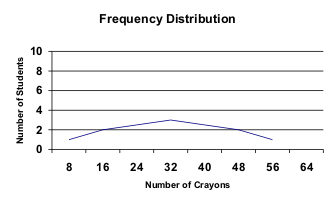Statistics: An Introduction

# Analyzing Individual Variables

The statistical procedures used to analyze a single variable describing a group (such as a population or representative sample) involve measures of central tendency and measures of variation. To explore these measures, a researcher first needs to consider the distribution, or range of values of a particular variable in a population or sample. Normal distribution occurs if the distribution of a population is completely normal. When graphed, this type of distribution will look like a bell curve; it is symmetrical and most of the scores cluster toward the middle. Skewed Distribution simply means the distribution of a population is not normal. The scores might cluster toward the right or the left side of the curve, for instance. Or there might be two or more clusters of scores, so that the distribution looks like a series of hills.

Once frequency distributions have been determined, researchers can calculate measures of central tendency and measures of variation. Measures of central tendency indicate averages of the distribution, and measures of variation indicate the spread, or range, of the distribution (Hinkle, Wiersma and Jurs 1988).

### Measures of Central Tendency

Central tendency is measured in three ways: mean, median and mode. The mean is simply the average score of a distribution. The median is the center, or middle score within a distribution. The mode is the most frequent score within a distribution. In a normal distribution, the mean, median and mode are identical.

 Student # of CrayonsA 8 B 16 C 16 D 32 E 32 F 32 G 48 H 48 J 56

### Measures of Variation

Measures of variation determine the range of the distribution, relative to the measures of central tendency. Where the measures of central tendency are specific data points, measures of variation are lengths between various points within the distribution. Variation is measured in terms of range, mean deviation, variance, and standard deviation (Hinkle, Wiersma and Jurs 1988).

The range is the distance between the lowest data point and the highest data point. Deviation scores are the distances between each data point and the mean.

Mean deviation is the average of the absolute values of the deviation scores; that is, mean deviation is the average distance between the mean and the data points. Closely related to the measure of mean deviation is the measure of variance.

Variance also indicates a relationship between the mean of a distribution and the data points; it is determined by averaging the sum of the squared deviations. Squaring the differences instead of taking the absolute values allows for greater flexibility in calculating further algebraic manipulations of the data. Another measure of variation is the standard deviation.

Standard deviation is the square root of the variance. This calculation is useful because it allows for the same flexibility as variance regarding further calculations and yet also expresses variation in the same units as the original measurements (Hinkle, Wiersma and Jurs 1988).